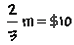Home    |    Teacher    |    Parents    |    Glossary    |    About UsAn equation is a mathematical sentence containing an equals sign. It tells us that two expressions mean the same thing, or represent the same number. An equation can contain variables and constants. Using equations, we can express math facts in short, easy-to-remember forms and solve problems quickly.

Here are several examples of equations. You can think of the letters as containers, or boxes, that can hold different numbers.

Example 1

3z + 2 = 14       x - 9 = 20       p + 2p = 3

The most important skill to develop in algebra is the ability to translate a word problem into the correct equation, so that you can solve the problem easily. Let's try a few examples:

Example 2

A number n times 3 is equal to 120.

This is an easy one. The word "times" tells you that you must multiply the variable n by 3, and that the result is equal to 120. Here's how to write this equation:

3n = 120

Here's one that's a little trickier.

Example 3.

Ten dollars was two-thirds of the total money spent.

What are we trying to find in this statement? The unknown amount is the total money spent. Let's call this m. We know that ten dollars is equal to two-thirds of m, so we can write the equation like this:Example 4.

Tim worked for 7 hours on Saturday and mowed 3 lawns. How much time, on average, did he spend on each lawn?

Let the letter "t" represent the average time per lawn, the unknown value. Then, 3t  would represent the time to mow all three lawns, and we know that this is equal to 7 hours. We can write the equation like this:

3t = 7 hours

 Homework Help | Algebra | The Language of AlgebraEmail this page to a friendSearch·  Definitions·  Order of Operations·  Writing equations·  Writing inequalitiesFirst Glance In Depth Examples WorkoutWriting equations